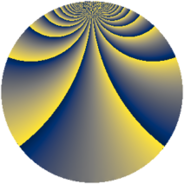# Properties

 Label 1805.2.lLevel $1805$ Weight $2$ Character orbit 1805.l Rep. character $\chi_{1805}(293,\cdot)$ Character field $\Q(\zeta_{12})$ Dimension $616$ Sturm bound $380$

# Related objects

## Defining parameters

 Level: $$N$$ $$=$$ $$1805 = 5 \cdot 19^{2}$$ Weight: $$k$$ $$=$$ $$2$$ Character orbit: $$[\chi]$$ $$=$$ 1805.l (of order $$12$$ and degree $$4$$) Character conductor: $$\operatorname{cond}(\chi)$$ $$=$$ $$95$$ Character field: $$\Q(\zeta_{12})$$ Sturm bound: $$380$$

## Dimensions

The following table gives the dimensions of various subspaces of $$M_{2}(1805, [\chi])$$.

Total New Old
Modular forms 840 744 96
Cusp forms 680 616 64
Eisenstein series 160 128 32

## Trace form

 $$616 q + 6 q^{2} + 6 q^{3} + 6 q^{5} + 8 q^{6} + 16 q^{7} + O(q^{10})$$ $$616 q + 6 q^{2} + 6 q^{3} + 6 q^{5} + 8 q^{6} + 16 q^{7} + 6 q^{10} + 8 q^{11} + 6 q^{13} + 24 q^{15} + 204 q^{16} - 8 q^{17} - 88 q^{20} - 12 q^{21} - 6 q^{22} + 22 q^{23} + 6 q^{25} - 64 q^{26} - 36 q^{28} - 28 q^{30} + 36 q^{32} - 24 q^{35} - 64 q^{36} + 72 q^{40} + 48 q^{41} - 30 q^{42} - 14 q^{43} + 16 q^{45} - 26 q^{47} - 48 q^{48} - 60 q^{51} + 18 q^{52} - 12 q^{53} + 4 q^{55} - 4 q^{58} - 114 q^{60} - 36 q^{61} - 4 q^{62} - 20 q^{63} + 116 q^{66} - 18 q^{67} + 124 q^{68} - 18 q^{70} + 12 q^{71} - 132 q^{72} + 20 q^{73} - 128 q^{77} - 84 q^{78} - 62 q^{80} + 48 q^{81} - 32 q^{82} + 20 q^{83} + 74 q^{85} - 72 q^{86} + 100 q^{87} - 72 q^{90} + 84 q^{91} - 168 q^{92} - 24 q^{93} - 64 q^{96} + 72 q^{98} + O(q^{100})$$

## Decomposition of $$S_{2}^{\mathrm{new}}(1805, [\chi])$$ into newform subspaces

The newforms in this space have not yet been added to the LMFDB.

## Decomposition of $$S_{2}^{\mathrm{old}}(1805, [\chi])$$ into lower level spaces

$$S_{2}^{\mathrm{old}}(1805, [\chi]) \cong$$ $$S_{2}^{\mathrm{new}}(95, [\chi])$$$$^{\oplus 2}$$